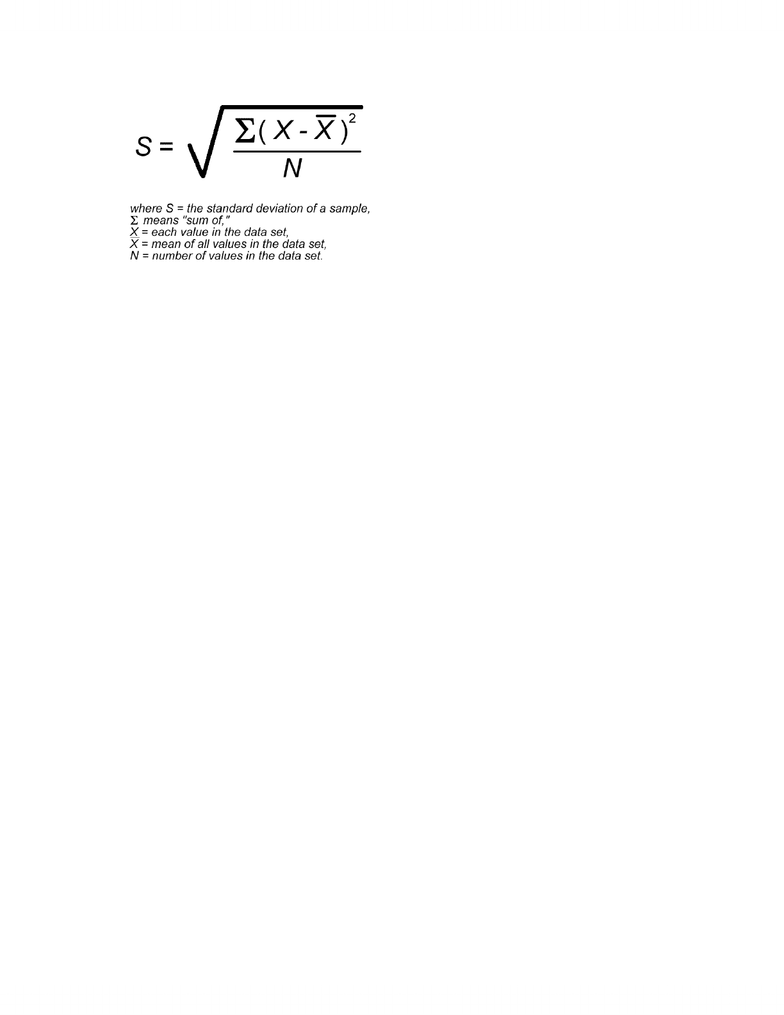Study Guides (390,000)
CA (150,000)
U of G (8,000)
SOAN (200)
Final

SOAN 2120 Study Guide - Final Guide: Squared Deviations From The Mean, Null Hypothesis, Standard Deviation

Department
Sociology and Anthropology
Course Code
SOAN 2120
Professor
David Walters
Study Guide
Final

This preview shows page 1. to view the full 5 pages of the document.Lecture Note Information
Purpose of the Course:
The purpose of this course is to provide an introduction to research methodology in the
social sciences. This class will cover both qualitative and quantitative approaches to
social research.
Quantitative Vs. Qualitative:
Quantitative applied statistics, numbers
Qualitative interviews, research, everything else
Experiments Vs. Surveys:
Experiments very controlled environment Pretest, Treatment, Post-test
Survey ask them a number of q‟s – do not manipulate subjects each question on
survey represents a variable
Types of Variables:
Quantitative Variables continuous (rank), numerical
(e.g. education… #of years of schooling and income…# money)
Categorical non-rank order, non-numerical
(e.g. religion… Catholics do not rank higher than protestants and race…skin colour)
Distributions:
When the mean is equal to the median (the average is equal to the middle), this is a
normal distribution.
Normal distributions allow us to conduct significance tests.
The distribution of a variable using data from our sample is called sample distribution.
The distribution of the variable in the population is called population distribution.
The distribution of the mean of a variable from all possible samples is the sampling
distribution.
Sampling distribution as its standard deviation gets smaller as the size of the sample get
larger.
Sampling distribution based on calculating a statistic over and over again from sample
samples of the same size.
Measures of Central Tendency:
Mean, Mode, Median, Range
Refresher: mean = average, mode = most occurring, median = middle (when written out
from least to greatest), and range = largest number subtracted by smallest number
Variance/Standard Deviation:
You're Reading a Preview

Unlock to view full version

Only page 1 are available for preview. Some parts have been intentionally blurred.(N = so in other words, the sample size)
Standard deviation is the square root of the variance.
To calculate the standard deviation:
1) calculate the mean
2) for every observation:
a. first observation minus mean = value. Square that value = new squared
value.
b. Second observation minus mean = value. Square that value = new squared
value.
c.
d.
e. last observation minus mean = value. Square that value = new squared
VALUE.
3) Add all the squared values to new a new value (sum of squared deviations).
4) Divide that value by the sample size (n) minus 1.
Validity and Reliability:
(Kayley go to Brad‟s notes for this – UoG notebook)
Unbiased/Sampling Error:
The difference between the statistic and the parameter due to random process is known as
sampling error.
Random samples have less error than nonprobability samples. The sampling error
decreases as sample size goes up.
Central Limit Theory:
- regardless of the population distribution, with repeated sampling, the shape of the
sampling distribution is approximately „normal‟ (or bell shaped) with the
population parameter at its centre.
- ??
Law of Large Numbers:
- the larger the samples, the more the confidence because it‟s closer to the
population estimate
Z-Scores
- z scores = take „x‟, subtract the mean and divide by the standard deviation
You're Reading a Preview

Unlock to view full version